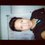# 1+n = n+1 Well-Ordering Principle

Can u help with understanding how proof that $$1+n = n+1$$ using the well-ordering property?

The way that i take to solve it, is the following: Set $A= \{ n \in \N | 1+n \ne n+1 \}$ , $A \ne \oslash$ . and $m$ is, by the well ordering principle (WOP), the least element of $A$ such that $m < n$ .

Now, being $m-1 < m < n$ that satisfy

$1+ (m-1) = (m-1) + 1 \implies m = m$

Showing that $m$ is not the least element of $A$ in this way:

$\forall n < m \implies 1+n = n+1$

In this sense, a contradiction is reached with the assumption that

$A \ne \oslash$

Sorry for see this "horror logic math", im a beginner.Note by Chris Juarez
9 months ago

This discussion board is a place to discuss our Daily Challenges and the math and science related to those challenges. Explanations are more than just a solution — they should explain the steps and thinking strategies that you used to obtain the solution. Comments should further the discussion of math and science.

When posting on Brilliant:

• Use the emojis to react to an explanation, whether you're congratulating a job well done , or just really confused .
• Ask specific questions about the challenge or the steps in somebody's explanation. Well-posed questions can add a lot to the discussion, but posting "I don't understand!" doesn't help anyone.
• Try to contribute something new to the discussion, whether it is an extension, generalization or other idea related to the challenge.

MarkdownAppears as
*italics* or _italics_ italics
**bold** or __bold__ bold
- bulleted- list
• bulleted
• list
1. numbered2. list
1. numbered
2. list
Note: you must add a full line of space before and after lists for them to show up correctly
paragraph 1paragraph 2

paragraph 1

paragraph 2

[example link](https://brilliant.org)example link
> This is a quote
This is a quote
    # I indented these lines
# 4 spaces, and now they show
# up as a code block.

print "hello world"
# I indented these lines
# 4 spaces, and now they show
# up as a code block.

print "hello world"
MathAppears as
Remember to wrap math in $$ ... $$ or $ ... $ to ensure proper formatting.
2 \times 3 $2 \times 3$
2^{34} $2^{34}$
a_{i-1} $a_{i-1}$
\frac{2}{3} $\frac{2}{3}$
\sqrt{2} $\sqrt{2}$
\sum_{i=1}^3 $\sum_{i=1}^3$
\sin \theta $\sin \theta$
\boxed{123} $\boxed{123}$

Sort by:

I like the idea of this proof. It looks to prove a commutative property of the addition of natural numbers using the WOP.

I think the strategy for this kind of proof is to say m is the least element of $\bold{A}$ then show that this would imply that $p$, a smaller number than $m$ e.g. $m-1$ also had to be in $\bold{A}$. You would show that $1+p$ does not equal $p+1$. This is a contradiction so $\bold{A}$ is an empty set proving the theorem.

lemma: $1-1 = (-1)+1$ (to be proven somehow)

$1+m \neq m+1$ (because $m$ is an element of $\bold{A}$)

$\therefore 1+m-1 \neq m+1-1$ (subtract $1$ from both sides)

$\therefore 1+p \neq m-1+1$ (from $p=m-1$ and lemma)

$\therefore 1+p \neq p+1$ (a contradiction as $p < m$)

It might help to explicitly state the base case for $n=1$, i.e. that $1+1=1+1$. This rules out the possibility that $m=1$ and $p = m-1$ is not a natural number.

I think the $1+(m-1)=(m-1)+1⟹m=m$ line in your proof is the most interesting. I think this implication assumes the associative property of addition. Associativity may be enough to prove this commutative property without invoking the WOP: e.g. $1+((n-1)+1)=1+n$ but, assuming associativity, this also equals $(1+(n-1))+1$ which equals $n+1$.

- 7 months, 3 weeks ago

Hi Unai, thanks for your comment.

I think it is correct as it is written, not a typo. I would be interested in your thoughts.

Of course in the real world 1+p does equal p+1 but in the strange world where there are natural numbers in A (the set of natural numbers where 1+n does not equal n+1), the task is to show that the idea of a least element in A does not make sense because for every least element m, there is a lesser element p that also meets the criteria for membership of A. Because we know that natural numbers obey the WOP, this absence of a true least element means that A is in fact empty and this proves this commutative property of addition.

Not all "addition" is commutative. If we expand the idea of addition to include actions like concatenating stings of characters then "1"+"n"="1n"and "n"+"1"="n1". The task is to prove that addition of natural numbers is commutative like we think it should be.

- 7 months, 1 week ago

Thank you so much for the explanation, I got a bit confused but rereading with a more structured vision really helped. The proof from OP confused me quite a bit and it doesn't help that my education level atm is still quite low. Thanks :)

- 7 months, 1 week ago

The solution is great, but I think there's a small typo: in the last line of the reasoning shouldn't it be 1+p not equal to p ?

- 7 months, 1 week ago